# Three Phase Horizontal Separator Sizing

For sizing a horizontal three-phase separator it is necessary to specify a vessel diameter and a seam-to-seam vessel length. The gas capacity and retention time considerations establish certain acceptable combinations of diameter and length. The need to settle 500-micron water droplets
from the oil establishes a maximum diameter.

Gas Capacity
The gas capacity constraints provide the following formula,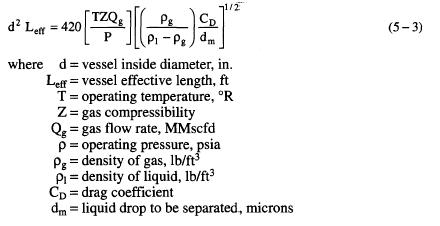Retention Time
Retention time constraints give another equation that provides acceptable combinations of d and Leff.where Qw = water flow rate, bpd
(tr)w – water retention time, min
Q0 = oil flow rate, bpd
(t.r)0 = oil retention time, min
Derivation of Equation 5-4
t is in S, Vol in ft3, Q in ft3/s, D in ft, d in inches, Leff in ftSettling Equation
The requirement that 500-micron water droplets be capable of settling out of the oil pad establishes a maximum oil pad thickness given by the following formula:This is the maximum thickness the oil pad can be and still allow the water droplets to settle out in time (t.r)0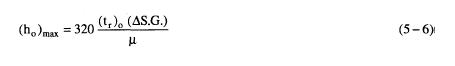For a given oil retention time and a given water retention time the maximum oil pad thickness constraint establishes a maximum diameter in accordance with the following procedure:
1. Compute (h0)max.
2. Calculate the fraction of the vessel cross-sectional area occupied by
the water phase. This is given by: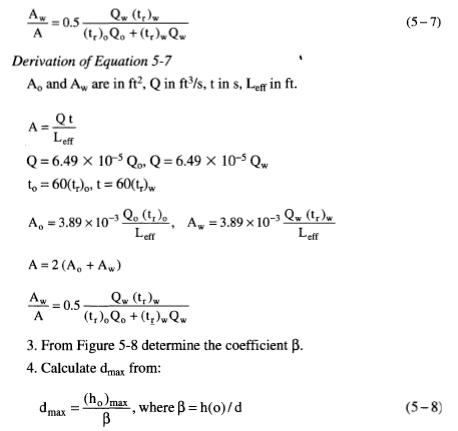Any combination of d and Leff that satisfies all three of Equations 5-3,
5-4, and 5-5 will meet the necessary criteria.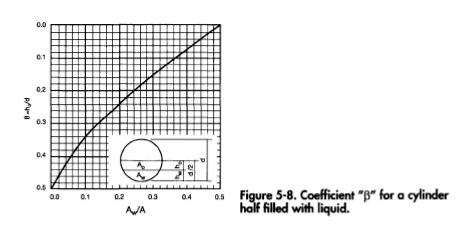Coefficient "|3" for a cylinder half filled with liquid.

Seam-to-Seam Length and Slenderness Ratios
The seam-to-seam length can be estimated from the effective length using the same formulas as for two-phase separators. Where the gas capacity governs, the slenderness ratio should be limited to less than 4 or 5 to prevent re-entrainment of liquid at the gas-liquid interface. If the separator sizing is based on liquid capacity a higher slenderness ratio is acceptable. There is the possibility of generating internal waves at the oil-water interface. Unless a more elaborate study is performed, it is recommended that slenderness ratios of less than 4 be chosen. Most common horizontal three-phase separators have slenderness ratios between 3 and 5.

Procedure for Sizing Three-Phase Horizontal Separators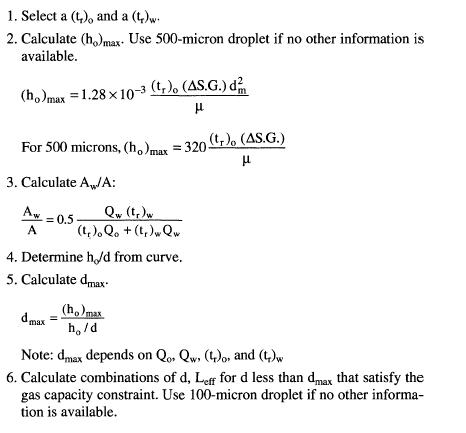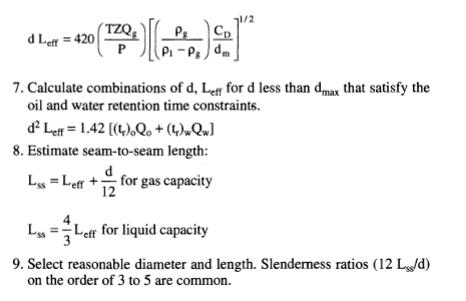For separators other than 50% full of liquid, equations can be derived similarly, using the actual oil and water areas. The equations are derived using the same principles.